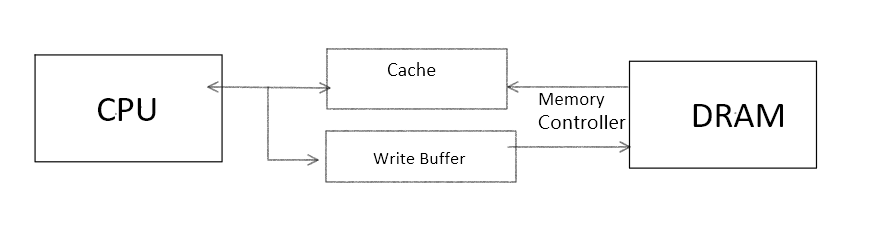## 写命中

### 写直达(Write Through)Write Buffer是一个FIFO队列，一般只有4字节。

### 写回(Write Back)

``public class Padding {      private static class X {         public long p1,p2,p3,p4,p5,p6,p7;//cache padding         public volatile long x=0L;         public long p9,p10,p11,p12,p13,p14,p15;         //8*8刚好占满Cache一行，p9...p15只是为了确保x单独在一行中，不与其他频繁修改的变量在一起     }     public static X[] arrX=new X;     static {         arrX=new X();         arrX=new X();     }     public static void main(String[] args) throws InterruptedException {         Thread thread1=new Thread(()->{             for(long i=0;i<100_000_000L;i++)                 arrX.x=i;         });          Thread thread2=new Thread(()->{             for(long i=0;i<100_000_000L;i++)                 arrX.x=i;         });         final long start=System.nanoTime();         thread1.start();         thread2.start();         thread1.join();         thread2.join();         System.out.println((System.nanoTime()-start)/1_000_000);     } } ``
``package mytask;  public class NoPadding {     private static class X {         public volatile long x=0L;     }      public static X[] arrX=new X;     static {         arrX=new X();         arrX=new X();     }      public static void main(String[] args) throws InterruptedException {         Thread thread1=new Thread(()->{             for(long i=0;i<100_000_000L;i++)                 arrX.x=i;         });          Thread thread2=new Thread(()->{             for(long i=0;i<100_000_000L;i++)                 arrX.x=i;         });         final long start=System.nanoTime();         thread1.start();         thread2.start();         thread1.join();         thread2.join();         System.out.println((System.nanoTime()-start)/1_000_000);     } } ``

## 写不命中